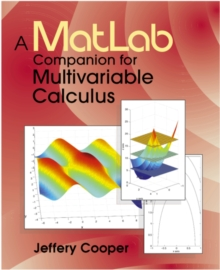# A MATLAB COMPANION FOR MULTIVARIABLE CALCULUS

by: Cooper, Jeffery
Format: Paperback

### price comparison#### Description

Product Description Offering a concise collection of MatLab programs and exercises to accompany a third semester course in multivariable calculus, A MatLab Companion for Multivariable Calculus introduces simple numerical procedures such as numerical differentiation, numerical integration and Newton's method in several variables, thereby allowing students to tackle realistic problems. The many examples show students how to use MatLab effectively and easily in many contexts. Numerous exercises in mathematics and applications areas are presented, graded from routine to more demanding projects requiring some programming. Matlab M-files are provided on the Harcourt/Academic Press web site at http://www.harcourt-ap.com/matlab.html. Review Excerpts from the reviews: "This text does a good job of explaining how MATLAB works at exactly the appropriate level. The use of MATLAB also encourages students toward more numerical thinking than do the packages that are primarily symbolic manipulations." "The author's writing is very clear. Students should find it pleasant reading, as I did." "I liked the problems. They presented the main issues and calculations from a variety of angles, so that students have to think and learn on almost all of them." "I like the serious applications at the end-to fluids, for example: this would also be helpful for nice demonstrations which the instructor could do in class." "My favorite aspect of this text is that by using MATLAB it naturally incorporates more numerical thinking into Multivariable Calculus. This builds on recent trends in Single-Variable Calculus 'reform'." From the Back Cover A MATLAB Companion for Multivariable Calculus Jeffery Cooper, University of Maryland This book provides a concise collection of MATLAB programs and exercises to accompany a course in multivariable calculus. The main ideas of calculus in several variables are presented alongside examples of computations and graphics displays using the MATLAB computer algebra software. The book's many examples demonstrate how to use MATLAB effectively and easily in a variety of contexts. Simple numerical procedures are introduced -- such as numerical differentiation, numerical integration and Newton's method in several variables -- allowing students to tackle problems utilizing these techniques and optimizing the relevant MATLAB applications. The author provides numerous examples of short codes which students can then modify to solve problems. Exercises contained in this volume include applications to problems across disciplines, such as economics, physics, engineering and biology. Supplementary MATLAB files are provided on the publisher's Web site* to implement some graphical displays too complex for programming by students new to the software. These files can be used by students or can also serve as practical MATLAB classroom demonstrations for instructors teaching the course. * http://www.harcourt-ap.com/mathematics.html (title entry under "Calculus") Exerpts from the reviews: "This text does a good job of explaining how MATLAB works at exactly the appropriate level. The use of MATLAB also encourages students toward more numerical thinking than do the packages that are primarily symbolic manipulations." "The author's writing is very clear. Students should find it pleasant reading, as I did." "I liked the problems. They presented the main issues and calculations from a variety of angles, so that students have to think and learn on almost all of them." "I like the serious applications at the end-to fluids, for example: this would also be helpful for nice demonstrations which the instructor could do in class." "My favorite aspect of this text is that by using MATLAB it naturally incorporates more numerical thinking into Multivariable Calculus. This builds on recent trends in Single-Variable Calculus 'reform'."| A MATLAB Companion for Multivariable Calculus Jeffery Cooper, University of Maryland This book provides a concise colle

#### Details

Product Code: 9780121876258
ISBN: 012187625X
Publisher: Academic Press
Publication Date: 2001-01-01
Number of Pages: 312 pages
Languages: english
Edition: 1
Dimension: 7.28 x 0.59 x 9.09 inches
Shipping Weight: 1.1 pounds